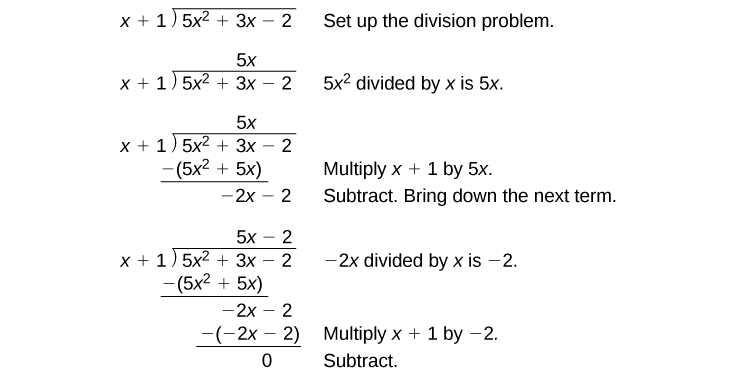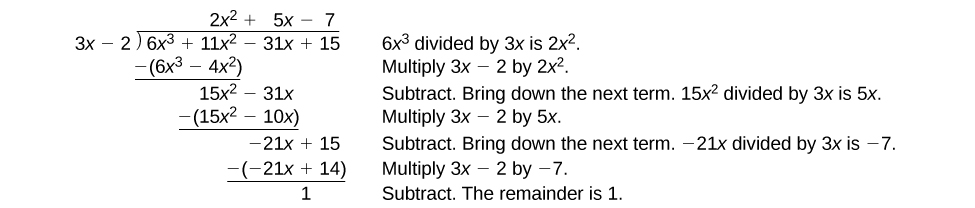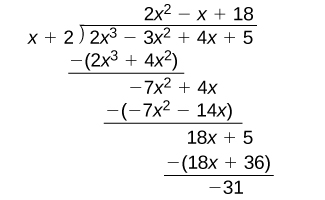# 5.4 Dividing polynomials  (Page 2/6)

 Page 2 / 6

Given a polynomial and a binomial, use long division to divide the polynomial by the binomial.

1. Set up the division problem.
2. Determine the first term of the quotient by dividing the leading term of the dividend by the leading term of the divisor.
3. Multiply the answer by the divisor and write it below the like terms of the dividend.
4. Subtract the bottom binomial    from the top binomial.
5. Bring down the next term of the dividend.
6. Repeat steps 2–5 until reaching the last term of the dividend.
7. If the remainder is non-zero, express as a fraction using the divisor as the denominator.

## Using long division to divide a second-degree polynomial

Divide $\text{\hspace{0.17em}}5{x}^{2}+3x-2\text{\hspace{0.17em}}$ by $\text{\hspace{0.17em}}x+1.$The quotient is $\text{\hspace{0.17em}}5x-2.\text{\hspace{0.17em}}$ The remainder is 0. We write the result as

$\frac{5{x}^{2}+3x-2}{x+1}=5x-2$

or

$5{x}^{2}+3x-2=\left(x+1\right)\left(5x-2\right)$

## Using long division to divide a third-degree polynomial

Divide $\text{\hspace{0.17em}}6{x}^{3}+11{x}^{2}-31x+15\text{\hspace{0.17em}}$ by $\text{\hspace{0.17em}}3x-2.\text{\hspace{0.17em}}$There is a remainder of 1. We can express the result as:

$\frac{6{x}^{3}+11{x}^{2}-31x+15}{3x-2}=2{x}^{2}+5x-7+\frac{1}{3x-2}$

Divide $\text{\hspace{0.17em}}16{x}^{3}-12{x}^{2}+20x-3\text{\hspace{0.17em}}$ by $\text{\hspace{0.17em}}4x+5.\text{\hspace{0.17em}}$

$4{x}^{2}-8x+15-\frac{78}{4x+5}$

## Using synthetic division to divide polynomials

As we’ve seen, long division of polynomials can involve many steps and be quite cumbersome. Synthetic division is a shorthand method of dividing polynomials for the special case of dividing by a linear factor whose leading coefficient is 1.

To illustrate the process, recall the example at the beginning of the section.

Divide $\text{\hspace{0.17em}}2{x}^{3}-3{x}^{2}+4x+5\text{\hspace{0.17em}}$ by $\text{\hspace{0.17em}}x+2\text{\hspace{0.17em}}$ using the long division algorithm.

The final form of the process looked like this:There is a lot of repetition in the table. If we don’t write the variables but, instead, line up their coefficients in columns under the division sign and also eliminate the partial products, we already have a simpler version of the entire problem.Synthetic division carries this simplification even a few more steps. Collapse the table by moving each of the rows up to fill any vacant spots. Also, instead of dividing by 2, as we would in division of whole numbers, then multiplying and subtracting the middle product, we change the sign of the “divisor” to –2, multiply and add. The process starts by bringing down the leading coefficient.We then multiply it by the “divisor” and add, repeating this process column by column, until there are no entries left. The bottom row represents the coefficients of the quotient; the last entry of the bottom row is the remainder. In this case, the quotient is $\text{\hspace{0.17em}}2{x}^{2}–7x+18\text{\hspace{0.17em}}$ and the remainder is $\text{\hspace{0.17em}}–31.\text{\hspace{0.17em}}$ The process will be made more clear in [link] .

## Synthetic division

Synthetic division is a shortcut that can be used when the divisor is a binomial in the form $\text{\hspace{0.17em}}x-k\text{\hspace{0.17em}}$ where $\text{\hspace{0.17em}}k\text{\hspace{0.17em}}$ is a real number. In synthetic division    , only the coefficients are used in the division process.

Given two polynomials, use synthetic division to divide.

1. Write $\text{\hspace{0.17em}}k\text{\hspace{0.17em}}$ for the divisor.
2. Write the coefficients of the dividend.
3. Bring the lead coefficient down.
4. Multiply the lead coefficient by $\text{\hspace{0.17em}}k.\text{\hspace{0.17em}}$ Write the product in the next column.
5. Add the terms of the second column.
6. Multiply the result by $\text{\hspace{0.17em}}k.\text{\hspace{0.17em}}$ Write the product in the next column.
7. Repeat steps 5 and 6 for the remaining columns.
8. Use the bottom numbers to write the quotient. The number in the last column is the remainder and has degree 0, the next number from the right has degree 1, the next number from the right has degree 2, and so on.

the third and the seventh terms of a G.P are 81 and 16, find the first and fifth terms.
if a=3, b =4 and c=5 find the six trigonometric value sin
pls how do I factorize x⁴+x³-7x²-x+6=0
in a function the input value is called
how do I test for values on the number line
if a=4 b=4 then a+b=
a+b+2ab
Kin
commulative principle
a+b= 4+4=8
Mimi
If a=4 and b=4 then we add the value of a and b i.e a+b=4+4=8.
Tariq
what are examples of natural number
an equation for the line that goes through the point (-1,12) and has a slope of 2,3
3y=-9x+25
Ishaq
show that the set of natural numberdoes not from agroup with addition or multiplication butit forms aseni group with respect toaaddition as well as multiplication
x^20+x^15+x^10+x^5/x^2+1
evaluate each algebraic expression. 2x+×_2 if ×=5
if the ratio of the root of ax+bx+c =0, show that (m+1)^2 ac =b^2m
By the definition, is such that 0!=1.why?
(1+cosA+IsinA)(1+cosB+isinB)/(cos@+isin@)(cos$+isin$)
hatdog
Mark
jaks
Ryan
how we can draw three triangles of distinctly different shapes. All the angles will be cutt off each triangle and placed side by side with vertices touching

#### Get Jobilize Job Search Mobile App in your pocket Now!ByByBy Jonathan LongBy Anh DaoByBy Rohini AjayBy OpenStaxBy Mahee BooBy OpenStaxBy Saylor FoundationBy Jams KaloBy Stephen Voron